# How to Vlookup multiple values in Excel with criteria

The tutorial shows a few ways to Vlookup multiple matches in Excel based on one or more conditions and return multiple results in a column, row or single cell.

When using Microsoft Excel for data analysis, you may often find yourself in situations when you need to get all matching values for a specific id, name, email address or some other unique identifier. An immediate solution that comes to mind is using the Excel VLOOKUP function, but the problem is that it can only return a single match.

Vlookup for multiple values can be done via a combined use of several functions. If you are far from being an Excel expert, don't hurry to leave this page. I will do my best to explain the underlying logic so that even a novice could understand the formulas and adjust them for solving similar tasks. Even better, I will show you another possible solution that takes just a few mouse clicks and does not require any knowledge of Excel formulas at all!

## How to do multiple Vlookup in Excel using a formula

As mentioned in the beginning of this tutorial, there is no way to make Excel VLOOKUP return multiple values. The task can be accomplished by using the following functions in an array formula:

• IF - evaluates the condition and returns one value if the condition is met, and another value if the condition is not met.
• SMALL - gets the k-th smallest value in the array.
• INDEX - returns an array element based on the row and column numbers you specify.
• ROW - returns the row number.
• COLUMN - returns the column number.
• IFERROR - traps errors.

Below you will find a few examples of such formulas.

### Formula 1. Vlookup multiple matches and return results in a column

Let's say, you have the seller names in column A and the products they sold in column B, column A containing a few occurrences of each seller. Your goal is to get a list of all products sold by a given person. To have it done, please follow these steps:

1. Enter a list of unique names in some empty row, in the same or another worksheet. In this example, the names are input in cells D2:G2: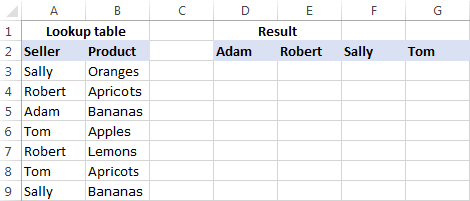Tip. To quickly get all different names in a list, you can use the UNIQUE function in Excel 365 or a more complex formula to extract distinct values in older versions.

2. Under the first name, select a number of empty cells that is equal to or greater than the maximum number of possible matches, enter one of the following array formulas in the formula bar, and press Ctrl + Shift + Enter to complete it (in this case, you will be able to edit the formula only in the entire range where it's entered). Or, you can enter the formula in the first cell, hit Ctrl + Shift + Enter, and then drag the formula down to a few more cells (in this case, you will be able to edit the formula in each cell individually).

`=IFERROR(INDEX(\$B\$3:\$B\$13, SMALL(IF(D\$2=\$A\$3:\$A\$13, ROW(\$B\$3:\$B\$13)-2,""), ROW()-2)),"")`

or

`=IFERROR(INDEX(\$B\$3:\$B\$13,SMALL(IF(D\$2=\$A\$3:\$A\$13,ROW(\$A\$3:\$A\$13)- MIN(ROW(\$A\$3:\$A\$13))+1,""), ROW()-2)),"")`

As you see, the 1st formula is a bit more compact, but the 2nd one is more universal and requires fewer modifications (we will elaborate more on the syntax and logic a bit further).

3. Copy the formula to other columns. For this, select the range of cells where you've just entered the formula, and drag the fill handle (a small square at the lower right-hand corner of the selected range) to the right.

The result will look something similar to this: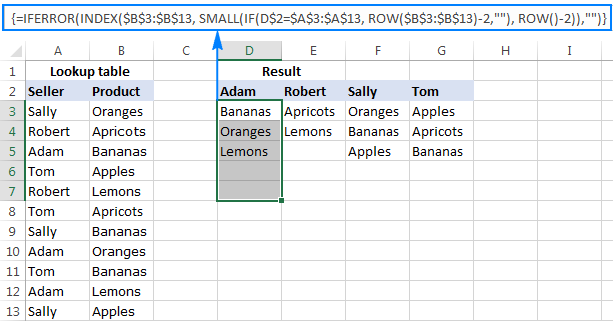#### How this formula works

This is an example of intermediate to advanced uses of Excel that implies basic knowledge of array formulas and Excel functions. Working from the inside out, here's what you do:

1. IF function

At the core of the formula, you use the IF function to get the positions of all occurrences of the lookup value in the lookup range: IF(D\$2=\$A\$3:\$A\$13, ROW(\$B\$3:\$B\$13)-2,"")

IF compares the lookup value (D2) with each value in the lookup range (A3:A13), and if the match if found, returns the relative position of the row; an empty string ("") otherwise.

The relative positions of the rows are calculated by subtracting 2 from ROW(\$B\$3:\$B\$13) so that the first row has position 1. If your return range begins in row 2, then subtract 1, and so on. The result of this operation is the array {1;2;3;4;5;6;7;8;9;10;11}, which goes to the value_if_true argument of the IF function.

Instead of the above calculation, you can use this expression: ROW(lookup_column)- MIN(ROW(lookup_column))+1, which returns the same result but does not require any changes regardless of the return column location. In this example, it'd be ROW(\$A\$3:\$A\$13)- MIN(ROW(\$A\$3:\$A\$13))+1.

So, at this point you have an array consisting of numbers (positions of matches) and empty strings (non-matches). For cell D3 in this example, we have the following array: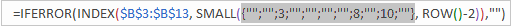If you check with the source data, you will see that "Adam" (lookup value in D2) appears on the 3rd, 8th and 10th positions in the lookup range (A3:A13).

2. SMALL function
Next, the SMALL(array, k) function steps in to determine which of the matches should be returned in a specific cell.

With array already established, let's work out the k argument, i.e. the k-th smallest value to be returned. For this, you make a sort of an "incremental counter" ROW()-n, where "n" is the row number of the first formula cell minus 1. In this example, we entered the formula in cells D3:D7, so ROW()-2 returns "1" for cell D3 (row 3 minus 2), "2" for cell D4 (row 4 minus 2), etc.

As the result, the SMALL function pulls the 1st smallest element of the array in cell D3, the 2nd smallest element in cell D4, and so on. And this transforms the initial long and complex formula into a very simple one, like this: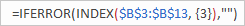Tip. To see the calculated value behind a certain part of the formula, select that part in the formula bar and press F9.

3. INDEX function

This part is easy. You use the INDEX function to return the value of an array element based on its row number.

4. IFERROR function

And finally, you wrap the formula in the IFERROR function to handle possible errors, which are inevitable because you cannot know how many matches will be returned for this or that lookup value, and therefore you copy the formula to a number of cells equal to or greater than the number of possible matches. Not to scare your users with a bundle of errors, simply replace them with an empty string (blank cell).

Note. Please notice the proper use of absolute and relative cell references in the formula. All references are fixed except for the relative column reference in the lookup value (D\$2), which should change based on a relative position of a column(s) where the formula is copied to return matches for other lookup values.

Putting all this together, we get the following generic formulas to Vlookup multiple values in Excel:

Formula 1:

IFERROR(INDEX(return_range, SMALL(IF(lookup_value = lookup_range, ROW(return_range )- m ,""), ROW() - n )),"")

Formula 2:

IFERROR(INDEX(return_range, SMALL(IF(lookup_value = lookup_range , ROW(lookup_range) - MIN(ROW(lookup_range ))+1,""), ROW() - n)),"")

Where:

• m is the row number of the first cell in the return range minus 1.
• n is the row number of the first formula cell minus 1.

Note. In the above example, both n and m are equal to "2" because our return range and formula range both begin in row 3. In your worksheets, these may be different numbers.

### Formula 2. Vlookup multiple matches and return results in a row

In case you want to return multiple values in rows rather than columns, change the above formulas this way:

`=IFERROR(INDEX(\$B\$3:\$B\$13, SMALL(IF(\$D3=\$A\$3:\$A\$13, ROW(\$B\$3:\$B\$13)-2,""), COLUMN()-4)),"")`

Or

`=IFERROR(INDEX(\$B\$3:\$B\$13,SMALL(IF(\$D3=\$A\$3:\$A\$13,ROW(\$A\$3:\$A\$13)- MIN(ROW(\$A\$3:\$A\$13))+1,""),COLUMN()-4)), "")`

Like in the previous example, both are array formulas, so remember to press the Ctrl + Shift + Enter shortcut to complete them correctly.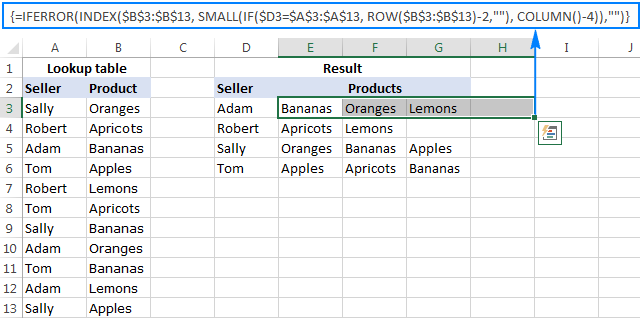The formulas work with the same logic as in the previous example, except that you use the COLUM function instead of ROW to determine which matching value should be returned in a specific cell: COLUMN()-n. Where n is the column number of the first cell where the formula is entered minus 1. In this example, the formula is input in cells E2:H2. With E being the 5th column, n is equal to "4" (5-1=4).

Note. For the formula to get copied correctly to other rows, mind the lookup value references, absolute column and relative row, like \$D3.

Wrapping up, here are the generic formulas for Vlookup with multiple results returned in rows:

Formula 1:

IFERROR(INDEX(return_range, SMALL(IF(lookup_value = lookup_range, ROW(return_range) - m, ""), COLUMN() - n)), "")

Formula 2:

IFERROR(INDEX(return_range, SMALL(IF(lookup_value = lookup_range, ROW(lookup_range) - MIN(ROW( lookup_range))+1,""),COLUMN() - n)), "")

Where:

• m is the row number of the first cell in the return range minus 1.
• n is the column number of the first formula cell minus 1.

### Formula 3. Vlookup multiple matches based on multiple conditions

You already know how to Vlookup for multiple values in Excel based on one condition. But what if you want to return multiple matches based on two or more criteria? Taking the previous examples further, what if you have an additional Month column, and you are looking to get a list of all products sold by a given seller in a specific month?

If you are familiar with arrays formulas, you may remember that they allow using asterisk (*) as the AND operator. So, you can just take the formulas discussed in the two previous examples, and have them check multiple conditions as demonstrated below.

#### Return multiple matches in a column

IFERROR(INDEX(return_range, SMALL(IF(1=((--(lookup_value1=lookup_range1)) * ( --(lookup_value2=lookup_range2))), ROW(return_range)-m,""), ROW()-n)),"")

Where:

• m is the row number of the first cell in the return range minus 1.
• n is the row number of the first formula cell minus 1.

Assuming the Seller list (lookup_range1) is in A3:A30, the Month list (lookup_range2) is in B3:B30, the seller of interest (lookup_value1) is in cell E3, and the month of interest (lookup_value2) is in cell F3, the formula takes the following shape:

`=IFERROR(INDEX(\$C\$3:\$C\$30, SMALL(IF(1=((--(\$E\$3=\$A\$3:\$A\$30)) * (--(\$F\$3=\$B\$3:\$B\$30))), ROW(\$C\$3:\$C\$30)-2,""), ROW()-2)),"")`

This layout may be useful for creating a dashboard, e.g. your users can enter a name in E3, month in F3 and get a list of products in column G: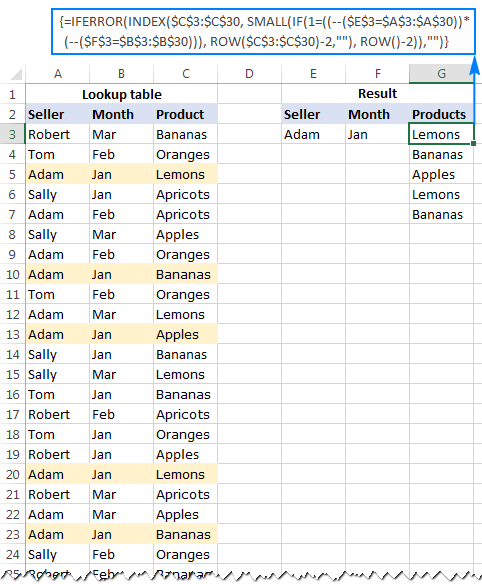#### Return multiple results in a row

If you want to pull multiple values based on multiple criteria sets, you may prefer the horizontal layout where results are returned in rows. In this case, use this following generic formula:

IFERROR(INDEX(return_range, SMALL(IF(1 = ((--(lookup_value1=lookup_range1)) * (--(lookup_value2 = lookup_range2))), ROW(return_range) - m, ""), COLUMN() - n)),"")

Where:

• m is the row number of the first cell in the return range minus 1.
• n is the column number of the first formula cell minus 1.

For our sample dataset, the formula goes as follows:

`=IFERROR(INDEX(\$C\$3:\$C\$30, SMALL(IF(1=((--(\$E3=\$A\$3:\$A\$30)) * (--(\$F3=\$B\$3:\$B\$30))), ROW(\$C\$3:\$C\$30)-2,""), COLUMN()-6)),"")`

And the result can resemble this: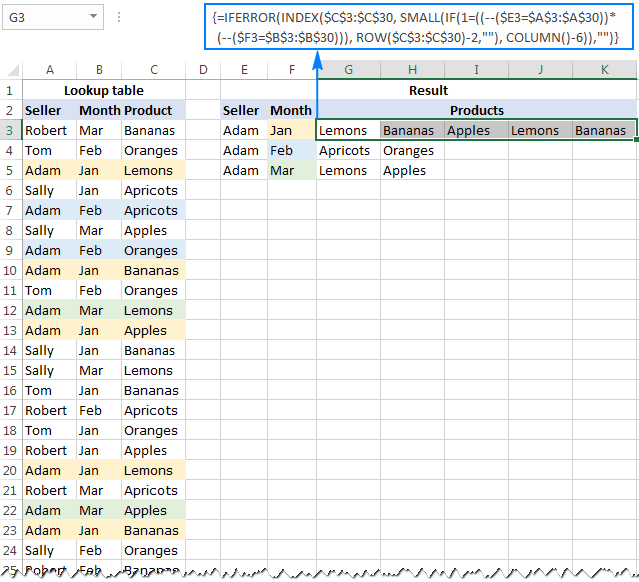In a similar manner, you can do multiple Vlookup with three, four or more conditions.

#### How these formulas work

Basically, the formulas to Vlookup multiple values with multiple conditions work with the already familiar logic, explained in the very first example. The only difference is that the IF function now tests multiple conditions:

1=((--(lookup_value1=lookup_range1))*(--(lookup_value2=lookup_range2))*…)

The result of each lookup_value=lookup_range comparison is an array of logical values TRUE (condition is met) and FALSE (condition is not met). The double unary operator (--) coerces the logical values into 1's and 0's. And because multiplying by zero always gives zero, in the resulting array, you have 1 only for those elements that meet all of the specified conditions. Now, you simply compare the final array with number 1 so that the ROW function returns the numbers of rows that meet all the conditions, an empty string otherwise.

A word of caution. All of the multiple Vlookup formulas discussed in this tutorial are array formulas. As such, each formula iterates through all elements of the arrays every time the source data is changed or the worksheet is recalculated. On large worksheets containing hundreds or thousands of rows, this may significantly slow down your Excel.

If you need to get matches from several sheets, use this guide: How to VLOOKUP across multiple sheets.

## How to Vlookup to return multiple values in one cell

I will be upfront - I don't know an easy way to lookup and return multiple matches in a single sell with formulas. However, I do know a formula-free (read "stress-free" :) way to do this by using two add-ins included with our Ultimate Suite for Excel. The detailed steps follow below.

#### Source data and expected result

As shown in the screenshot, we continue working with the dataset we've used in the previous example. But this time we want to achieve something different - instead of extracting multiple matches in separate cells, we want them to appear in a single sell, separated with a comma, space, or some other delimiter of your choosing.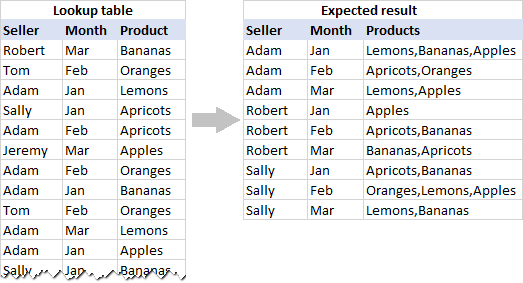#### Pull rows with multiple matches to the main table

In your main table, enter a list of unique names in the first column, months in the second column, and arrange them like shown in the screenshot below. After that, carry out the following steps:

1. Select your main table or click any cell within it, and then click the Merge Two Tables button on the ribbon: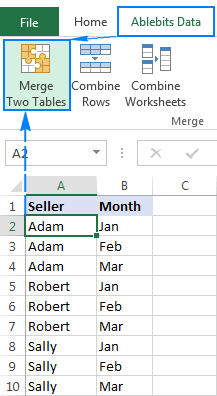2. The add-in is smart enough to identify and pick the entire table, so you just click Next: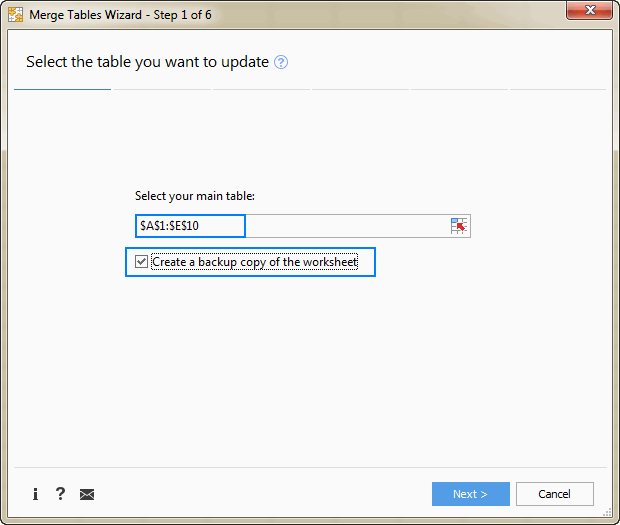Tip. When using the tool for the first time, it stands to reason to select the Create a backup copy of the worksheet box in case something goes wrong.

3. Select the lookup table, and click Next.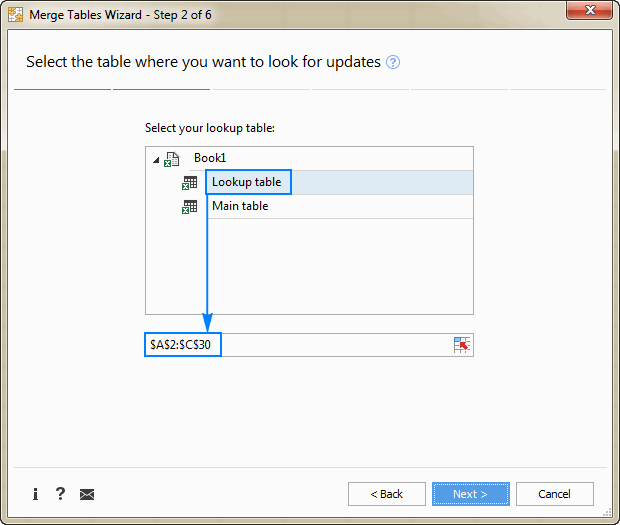4. Choose one or more matching pairs of columns that should be compared in the main table and lookup table (in this example, it's the Seller and Month columns), and then click Next.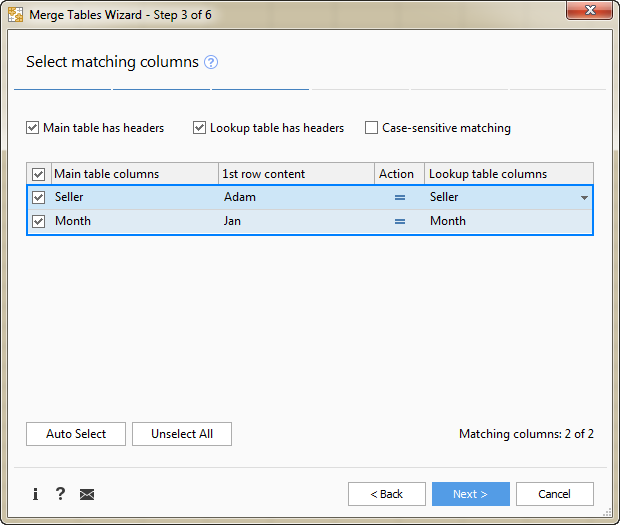5. Select the column(s) from which you want to pull matching values (Product in this example), and click Next.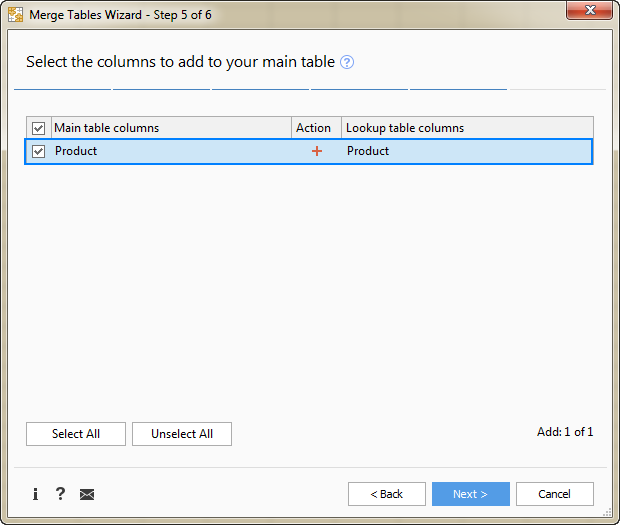6. Tell the add-in how exactly you want multiple matches to be arranged in the main table. For this example, we need the following option: Insert rows with duplicate matching values after the row with the same value. Make sure that no other option is selected and click Finish.At this point, you will have the following result - all matching rows are pulled to the main table and grouped by the values in the lookup columns - first by Seller, and then by Month: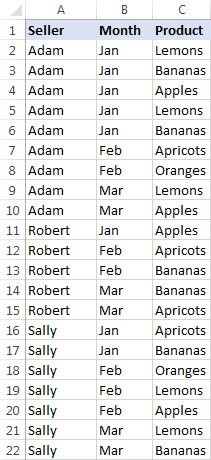The resulting table already looks nice, but it's not exactly what we wanted, right? As you remember, we are looking to Vlookup multiple matches and have them returned in a single sell, comma or otherwise separated.

#### Combine duplicates rows into one row

To merge "duplicate rows" in a single row, we are going to use another tool - Combine Rows Wizard.

1. Select the table produced by the Merge Tables tool (please see the screenshot above) or any cell within the table, and click the Combine Rows button on the ribbon: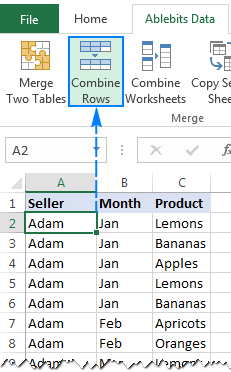2. Check if the add-in's got the table right, and click Next: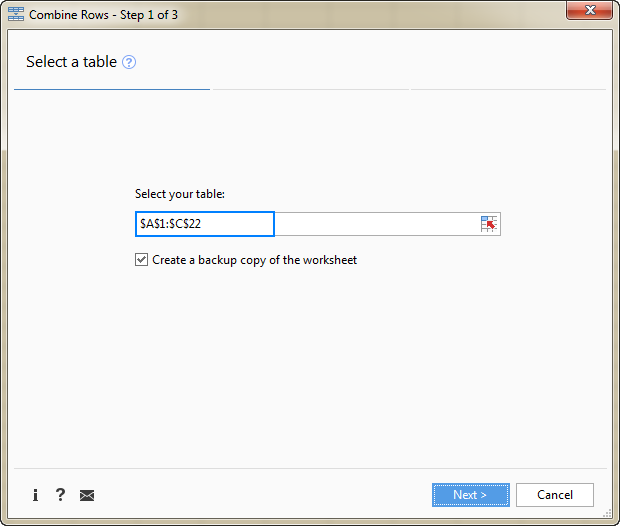3. Select the key column or columns (Seller and Month in this example), and click Next: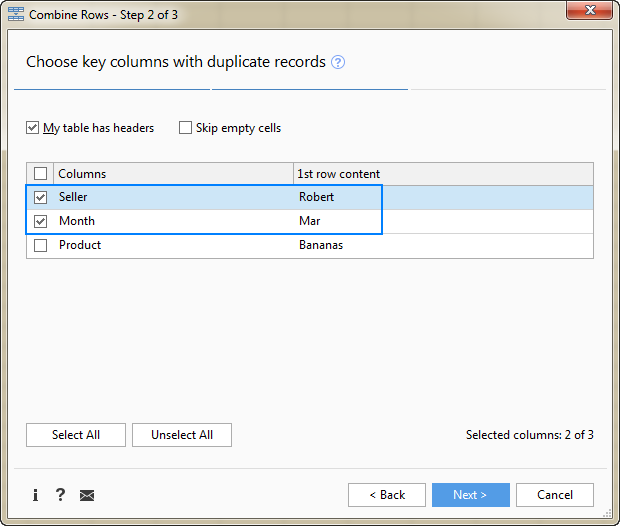4. Select the column(s) that contains multiple matches (Product in this example), choose the desired delimiter (semicolon, comma, space or line break), and click Finish.

Optionally, you can enable one of the additional features, or both:

• Delete duplicate values - if the column to be merged contains a few identical values, the first occurrence will be kept, duplicate matches will be deleted.

In this example, we do not check this option, and the add-in returns all found matches. For example, in cell C2, we have this string: Lemons, Bananas, Apples, Lemons, Bananas (please see the result on step 5 below). If you choose to delete duplicates, the result would be: Lemons, Bananas, Apples.

• Skip empty cells - self-explanatory :)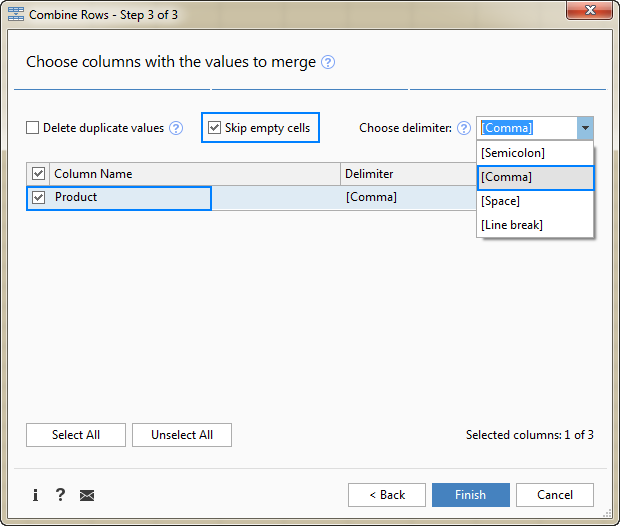5. Allow the add-in a few seconds for processing, and you are all set!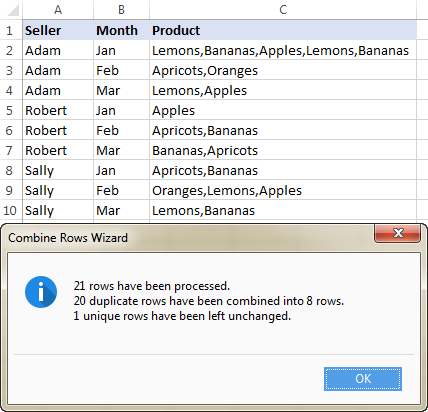This is how you can look up and return multiple values in Excel using our tools. If you are curious to give them a try, a fully-functional evaluation version of the Ultimate Suite is available for download below.

Vlookup Multiple Values - examples (.xlsx file)
Ultimate Suite - trial version (.exe file)

1. Please can you help me with retrieving values based on 2 different columns combination. In Sheet 1 I have 1 column with "name 1" and second column with "name 2" and a value column. In sheet 2 I want to look up if column 1 and column 2 match to retrieve me the value for that combination.

2. =IFERROR(INDEX(\$C\$2:\$C\$12,SMALL(IF(\$J2=\$B\$2:\$B\$12,ROW(\$B\$2:\$B\$12)- MIN(ROW(\$B\$2:\$B\$12))+1,""),COLUMN()-10)), "")

Can anyone help me to add 2 more conditions in this formula,
1- I don't want blank cells results "skip blank cells" (this formula is showing 0 for blank cells results),
2- I don't want same results in next cell/column if found similar in previous cell/column, cell content should not be repeated in next columns if found previously (this formula is finding cell content and repeating the same if found again in the list).

• Hello!
To get vlookup multiple values without duplicates, add the UNIQUE function to the formula:

=UNIQUE(IFERROR(INDEX(\$B\$3:\$B\$13, SMALL(IF(D\$2=\$A\$3:\$A\$13, ROW(\$B\$3:\$B\$13)-2,""), ROW(\$B\$3:\$B\$13)-2)),""))

3. Can someone help me on this?

for Example, I want products ABC1 (150Qty), XYZ1 (200Qty), PQR1 (100Qty), and there are multiple lots for each product (each lot has different available Qty) to buy which has below.
ABC1- 100(Avl Qty)
ABC1 - 20(Avl Qty)
ABC1 - 50(Avl Qty)

XYZ1- 150(Avl Qty
XYZ1 - 30(Avl Qty)
XYZ1 - 60(Avl Qty)

PQR1 - 40(Avl Qty)
PQR1 - 60(Avl Qty)
PQR1 - 50(Avl Qty)

In this case, I am looking for only whichever match with requested Qty like below result,
for ABC1, I want only 150Qty >= 100+20+30 (this 30come from 3rd lot)
for XYZ1, I want only 200Qty >= 150+30+20 (this 20come from 3rd lot)
for PQR1, I want only 100Qty >= 40+60 (3rd lot is not required since it is matched already (100=40+60))

• Hi!
Please try to enter the following formula in cell C2 and then copy it down along the column:

=IF(\$E\$1-SUM(\$B\$2:B2)>0,B2,\$E\$1-SUM(\$C\$1:C1))
\$E\$1 - desired quantity

• Thanks, it works but not exactly....

4. Hello together,

can someone help me? I am using the first formular "Formula 1. Vlookup multiple matches and return results in a column".
My problem is that I want to get multiple results not based on one Vlookup but for two.
If the first Vlookup or the second one is correct, I want to get the values. I have tried it with the "OR" formular but without succes.

I hope someone can help me.

• Maybe we can do it in the first example to make it more clear what I mean. My formular should check Adam OR Robert, and if he finds something it should give me the values.

• Hello!
Replace the OR operator with the sum of conditions. Try this formula -

=IFERROR(INDEX(\$B\$3:\$B\$13, SMALL(IF(1=(D\$2=\$A\$3:\$A\$13)+(E\$2=\$A\$3:\$A\$13), ROW(\$B\$3:\$B\$13)-2,"), ROW()-2),")

• Thank you very much!!! You are a genius :D

5. Hello together,

I have used the Formula 1. "Vlookup multiple matches and return results in a column" I wanted to extend it and add a or in the formular. But its not working.
I should get a result if the first or the second one matches. But it is not working. Can someone help me?

=IFERROR(INDEX(yoe2!\$D\$2:\$D\$666;Small(IF(OR(Vorlage!\$C\$3=yoe2!\$A\$2:\$A\$666;\$C\$2=yoe2!\$A\$2:\$A\$666);Row(yoe2!\$D\$2:\$D\$666)-1;"");Row()-9));"")

Thank you!

• Hi!
It is very difficult to understand a formula that contains unique references to your workbook worksheets. Hence, I cannot check its work, sorry. Explain in detail what you want to do and I will try to help.

• Hello Bellamy,

Your file is closed for editing, sorry.

Please follow the steps described in this tutorial to build the required formulas and obtain the data based on multiple conditions.

6. thanks i will try your suggestion!! thanks again!

7. hello, first of all thanks a lot for a very insightful article!

here is my question

my data is a bank statement wiht many different line items like this
16/09/2022 CARTE X1234 15/09 EAU VIVE -12.75
16/09/2022 PRELEVEMENT EUROPEEN 77012xxxxxx DE: BOUYGUES TELECOM ID: FR35ZZZ41xxxxx MOTIF: 09xxxxx142 -41.99
16/09/2022 PRELEVEMENT EUROPEEN 770120xxxxx DE: BOUYGUES TELECOM ID: FR35ZZZ41xxxxx MOTIF: 06xxxxx065 -27.99
15/09/2022 CARTE X567814/09 CAMAIEU 0136 -15.99
15/09/2022 CARTE X1234 14/09 Netflix.com COMMERCE ELECTRONIQUE -17.99
15/09/2022 CARTE X567814/09 FNAC -23
15/09/2022 CARTE X567814/09 CARREFOUR LABEGE -25.96
15/09/2022 CHEQUE 894 -292.5
14/09/2022 CARTE X1234 13/09 PECHERS SAUVAGES -27.13
14/09/2022 CARTE X1234 13/09 PICARD 1052 -66
13/09/2022 CARTE X5678REMBT 12/09 AMAZON EU SARL COMMERCE ELECTRONIQUE 9.73
13/09/2022 CARTE X1234 08/09 AMAZON PAYMENTS COMMERCE ELECTRONIQUE -49.98
12/09/2022 CARTE X1234 11/09 FOURNIL DE PIERRE -3.7
12/09/2022 CARTE X567809/09 PECHERS SAUVAGES -5.94
12/09/2022 CARTE X1234 10/09 PECHERS SAUVAGES -9.25
12/09/2022 CARTE X567810/09 AMAZON EU SARL COMMERCE ELECTRONIQUE -9.73
12/09/2022 CARTE X1234 10/09 DEUX CHAVANNESTF -11.58
12/09/2022 CARTE X1234 09/09 PECHERS SAUVAGES -15.71
12/09/2022 CARTE X567809/09 SAVEURS BIO -15.9
12/09/2022 CARTE X1234 10/09 DEUX CHAVANNE -21.56
12/09/2022 CARTE X1234 11/09 PECHERS SAUVAGES -24.02
12/09/2022 CARTE X567810/09 C DU CHATEAU -75
09/09/2022 CARTE X567808/09 PECHERS SAUVAGES -4.66

in a separate table i have manually attributed purchase Types with keyword that may appear inthe bank statement

EAU VIVE grocery
BOUYGUES TELECOM communications
CARREFOUR LABEGE grocery
Netflix.com entertainment
PECHERS SAUVAGES grocery
AMAZON EU SARL household
PICARD grocery
DEUX CHAVANNE grocery
CAMAIEU clothing

i want to do a VLOOKUP ( or similar) whereby i am searching for a keyword within the initial data, with a goal to add the defined TYPE based on the keyword. The challenge is that the actual source lookup value has a lot more text that the keyword in Table 2 ( the array).

the goal would be to end up w my final result like this

16/09/2022 CARTE X1234 15/09 EAU VIVE -12.75 grocery
16/09/2022 PRELEVEMENT EUROPEEN 77012xxxxxx DE: BOUYGUES TELECOM ID: FR35ZZZ41xxxxx MOTIF: 09xxxxx142 -41.99 communications
16/09/2022 PRELEVEMENT EUROPEEN 770120xxxxx DE: BOUYGUES TELECOM ID: FR35ZZZ41xxxxx MOTIF: 06xxxxx065 -27.99 communications
15/09/2022 CARTE X567814/09 CAMAIEU 0136 -15.99 clothing

etc

sorry i hope this is clear

thanks!

• Hello!
To search for a partial match, use the SEARCH function. Then use INDEX MATCH to find the matching value in the list.

=INDEX(\$I\$2:\$I\$10, MATCH(TRUE, ISNUMBER(SEARCH(\$H\$2:\$H\$10,A2)), 0))

Column H - keyword, column I - type. Column A - our data.

8. Hi,
If anyone can help me to create in and out trolley date time sheet. As i have done with just number id entry and it creates date time but when it return first time then its fine as it clocks right time. When the same number comes back then it creates return time from the column previous entry and puts same time as second in time. How i can create sequential entry of same id going out without entering so many entries.

9. Hi,

First, thank you for sharing!

Can you please provide a solution to the case below:

I have 3 columns (A: Containing reference information, B: Containing information to be searched, C: Search result)

Column A Column B Column C
File Name Name File Name, Document Name, Element Name, Name
Element ID Element Element ID, Element Name, Changed Element
Column Location
Document Name
Element Name
Name
Category
Warranty
Slope
Changed Element

What I need is to search in column A for any partial match with cell B2 (Name) or B3 (Element) and get the result in one cell,

• Hello!
To extract from column A the value that contains string from cell B2, use the INDEX MATCH formula:

=INDEX(A1:A10, MATCH(TRUE,ISNUMBER(SEARCH(B2,A1:A10)), 0))

Hope this is what you need.

10. Hello!

Firstly, I appreciate your effort in trying to help everyone who comments.

I was hoping you might be able to help write a formula to look for a designated value/name across a table, and where it is found (often on multiple rows), add together all associated number(s) in the adjacent cell(s), and display the product on a separate table.

For example, I have a table which lists recorded fuel costs for cars. Column A has car reference which may repeat for multiple entries ie car1, car2, car1, car3, car1. Column B has the cost associated with the entry. In this example, I referenced car1 having 3 separate cost incidents. I then want to make a table which combines costs for all cars ie in column A, car1 through car3, and in column B the total cost associated with each car.

I had been using Vlookup, but of course it only returns the first value for car1 in the above example and ignores the other 2 entries for car1.

Hopefully that makes sense! Much appreciated.

However, i have one need wherein I am checking two sheets (or you can call it basically two tables) wherein, one has more data than the other it has around 10Mn records and is bit inconsistent records. For example to present you a bit of complexity, I need help on how this can be approached. Below is a example table data.

Sheet B
item item# type method value
a 1 dog exp 1
a 1 cat exp 1
b 2 mat exp 1.1
b 2 dat std 1
b 2 fat exp 1
b 2 cat exp 0.8
b 2 dog exp 1.1
c 3 dat std 1
c 3 fat exp 1
d 4 fat exp 1.25
d 4 cat exp 1.1
d 4 dog exp 0.9
f 6 sat exp 1

Sheet A - This is master data

item item # type method value
a 1 dog exp 1.1
a 1 cat exp 1
a 1 bat exp 1
a 1 rat std 1.1
b 2 rat std 1
b 2 bat exp 1
b 2 mat exp 1
b 2 dat std 1.2
b 2 fat exp 0.8
b 2 cat exp 1.1
b 2 dog exp 1
b 2 yak exp 1
b 2 track std 1
b 2 owl exp 1
b 2 ram exp 1.25
b 2 sat exp 1
c 3 mat exp 0.9
c 3 dat std 1
c 3 fat exp 1
c 3 cat exp 0.83
d 4 fat exp 1.1
d 4 cat exp 1
d 4 dog exp 1
e 5 mat exp 1.09
f 6 sat exp 1.1
g 7 rat std 0.9
g 7 owl exp 0.83
g 7 ram exp 1
g 7 sat exp 1

What i see is if we do IFERROR and VLOOKUP and MATCH on the INDEX, we get the difference, but as we know this is row by row matching is not possible in this case, how can i take the delta of the above tables.

• Hello!
We have a ready-made solution for your task. Compare Tables for Excel searches for duplicates and unique values in any two tables. It is available as a part of our Ultimate Suite for Excel that you can install in a trial mode and check how it works for free.

• Thank you Alexander, is there another way apart from the ready-made free solution. The reason why i propose to have this is because business needs the solution as POC and we will be implementing this same on data model in sagemaker or other alike AI tool.
TA

12. Purchased items in bulk at different prices with different quantity on different dates.
Selling those items in retail.
Purchase sheet and sales sheets are different.
Both Sheets contain Item Code, Qty, Date and Rate

Want a solution to get purchase rate in front of sale until stock of that purchase ends.

• Hi!
Your task does not have a detailed description and cannot be solved with a single formula. If you have a specific question about the operation of a function or formula, I will try to answer it.

13. Hi, i have a question; i have 2 coloumns

A B
a x
b y
c z
a, b z, x
c, a y, z

How can i get results as;

a x z, x y, z
b y z, x
c z y, z

??
Pls help

• Thank you very much, i willl read them.

• Actually it's like
=IFERROR(INDEX(\$B\$3:\$B\$13,SMALL(IF(\$D3=\$A\$3:\$A\$13,ROW(\$A\$3:\$A\$13)- MIN(ROW(\$A\$3:\$A\$13))+1,""),COLUMN()-4)), "")
formula but i have to specify IF(\$D3=\$A\$3:\$A\$13 section as "look in coloumn A, whichever cell contains spesific text this is the reference one, continue formula" i cannot solve this

• Hi!
What do you want to calculate exactly? Your question is not entirely clear, please specify.

• Thank you for your concern, i am sorry for my english. İt is not fluent...

I want to see

A______B______
a______x,y____
b______z______
a______z, y____
c______x,z_____
b,a____x,y_____
c,b____x,y,z___
a,b,c__x,y,z___

as a result of:
D________E________F_________G________H
a________x,y______z,y________x,y______x,y,z
b________z________x,y________x,y,z____x,y,z
c________x,z______x,y,z_______x,y,z________

i cannot seperate A and B coloumn cells with =IFERROR(INDEX(\$B\$3:\$B\$13,SMALL(IF(\$D3=\$A\$3:\$A\$13,ROW(\$A\$3:\$A\$13)- MIN(ROW(\$A\$3:\$A\$13))+1,""),COLUMN()-4)), "") formula. It is static referenced.

• I told false, sorry.

This input :
A______B______
a______x,y____
b______z______
a______z, y____
c______x,z_____
b,a____x,y_____
c,b____x,y,z___
a,b,c__x,y,z___

should transform to this output:
D________E________F_________G________H
a________x,y______z,y________x,y______x,y,z
b________z________x,y________x,y,z____x,y,z
c________x,z______x,y,z_______x,y,z________

• Hi!
In C1, write the letter "a". Select the range D1:H1 and enter the array formula in the formula bar:

=IFERROR(INDEX(\$B\$1:\$B\$10, SMALL(IF(ISNUMBER(SEARCH(C1,\$A\$1:\$A\$10)), ROW(\$A\$1:\$A\$10)-0,""), COLUMN()-2)),"")

• Thank you very much for your answer. I will try this.

14. Hi, I want to Check something 1000 of Restricated Words From 1 line of Discription(200-300)Alphabet .Is there's any Formula to do that Please help Me in this. Thank you in advance

• Hello!
If you want to know if at least one word from the list occurs in a text, use the formula:

=SUM(IFERROR(SEARCH(B1:B1000,A1),0))>0

If you want to specify which words are found, try to enter the following formula in cell С1 and then copy it down along the column:

=ISNUMBER(SEARCH(B1,\$A\$1))

15. Hello thank you for the insights!

Btw, i'd like to ask about how to apply the array formula automatically througout certain range of cells?
It would be easier for me rather than dragging the formula all the way thank you.

16. I'm trying to work on a formula to look up the start date and rank of an employee in table 1 and then look up table 2 and if their start date is less than 12 months return a % based on their rank but if their start date is more than 12 months ago return a different % based on their rank.

Table 1 has start date, rack and % as column headers
Table 2 Has Ranking A B C D as headers, Rows are 'Before 9/02/2021' and 'after 09/02/2021'. The columns are filled in with various percentages in both rows.

I would like to look up the start date and rank in table 1 then look up table 2 and if the date on table 1 is 'before 9/02/2021' return the percentage from table 2 that matches the rank in the row 'before 09/02/2021' . If the date on table 1 is after 09/02/2021 return the percentage from table 2 that matches the rank in the row 'after 09/02/2021'

The date in the rows on table 2 are changing on a daily basis. As table 2 rows refer to either in the last 12 months or prior to 12 months.

I've tried a vlookup and match formulas but can't seem to get it to work. Any suggestions or better way to do this?

17. Can I use this across the wordsheet?
I mean the database in one sheet, and the result in other sheets.

I try but it did not work.

18. I have sets of data in 3 column and I need both values on column B & C to appear as an aswer when the answer is transposed:

APPLE ABC 123
APPLE BCD 231
APPLE CDE 321

APPLE ABC 123 BCD 231 CDE 321

is this possible so far my formula is this but I am only getting answer as: APPLE ABC BCD CDE

=INDEX(\$B\$2:\$B\$3650,SMALL(IF(\$F2=\$A\$2:\$A\$3650,ROW(\$A\$2:\$A\$3650)-ROW(\$A\$2)+1),COLUMN(A1)))

Thank you

• Hello!
To join multiple values into a string of text, use the TEXTJOIN function

=TEXTJOIN(" ",TRUE,IFERROR(INDEX(\$B\$2:\$C\$13, SMALL(IF(F\$2=\$A\$2:\$A\$13, ROW(\$A\$2:\$A\$13)-1,""), ROW(\$A\$2:\$A\$13)-2),{1,2}),""))

The formula works in any cell of 3 rows.

• Hi Alexander,

Thank you for looking into my query. Is it possible to get Location and Quantity below to appear in separate columns? With the original formula I could only extract location horizontally but I needed to find a way that the formula would return 2 values each time it finds unique reference is in column A and the answers I would like to get are in columns B and C and returning every instance horizontally in separate columns each time. Is this possible?

Produce Location Quantity
APPLE BOX123 50
ORANGE BOX124 70
APPLE BOX125 50
LEMON BOX125 60
ORANGE BOX123 60
APPLE BOX124 50

Produce Location Quantity Location Quantity Location Quantity
APPLE BOX123 50 BOX125 50 BOX124 50
ORANGE BOX124 70 BOX123 60
LEMON BOX125 60

Thank you very much.

• Hello!
Use a formula and get the result in the cell. Then replace the formula with its values using Paste Special.
Split strings in the cells by separators, as described in this guide.

19. Have you You Tube Channel?

20. Hi, thanks for this helpful post.
but i have a problem.
when my data is more than ~~3000, the formula dont work & shows empty cell.

• Hi!
Your question is not very clear. What formula are you using? What does 3000 mean?

21. I have a list of sales interactions by client, with several interaction dates on separate rows for each client name. I've successfully used Formula 2 to populate the interaction dates in a row from newest to oldest for each client. However, I would like to add rows to my interaction list for new interactions as they occur. This expands the range beyond what is currently in the formula (for instance, if my range is \$D\$2:\$D\$100, and I add a date for a new interaction into cell D101, it doesn't get captured). Is there a way to make the range of cells automatically update to include the newly added rows? Thanks!

• Hello!
Also, you can convert your data to Excel table and use structured references.
Dynamic named range and Excel spreadsheet will automatically grow when new data is added.

22. This information helped automate a process that has been plaguing my brain for the last 4 months. Thank you!

23. Hi,

I am using the same formula on the same dataset as you have it's just that the first table is in one file and the second one is in another file and it doesn't pick up. Please help.
Thank you.

Result: in file 1
Seller Month Product
Apricots
Oranges
Oranges

Actual Data: in file 2
Seller Month Product
Robert Mar-21 Bananas
Sally Jan-21 Apricots
Sally Mar-21 Oranges

24. I tried this using exactly the data in the example in Excel for Mac 16.51. It does not work correctly. In the test IF(D\$2=\$A\$3:\$A\$13, ROW(\$B\$3:\$B\$13)-2,"") the formula ROW(\$A\$3:\$A\$13) produces the same result regardless of the value of D2.

Maybe this is another instance of Excel for Mac and Excel for Windows being divergent.

• Hello

I have the same issue with a regular PC version, MS Office Professional Plus 2016.

Any suggestion?

• Hello!

I cannot say anything about Excel for Mac - did not have a chance to test the formulas there.

In my Excel 365, all the formulas work fine, exactly as described in this tutorial.

Tibor, you can downlaod our sample worsheet to check the behavior in your Excel.

25. This is almost exactly what I have been looking for. Is there a way to modify these formulas to work with a horizontal array instead of a vertical one?

Thanks

• I should add that this is the formula I would like to modify but have not been successful so far. =IFERROR(INDEX(\$B\$3:\$B\$13, SMALL(IF(D\$2=\$A\$3:\$A\$13, ROW(\$B\$3:
\$B\$13)-2,""), ROW()-2)),"")

Thanks

• Thanks, I'll give those a try

26. Is this applied for Excel 2007?
I applied the formula, only the first data came out, not multiple data.
Thank you.

• Hi Kaede,

Did you press Ctrl + Shift + Enter to complete the formula? In Excel 2007 - 2019, it only works as an array formula.

27. Hi, upon using the following formula,

=INDEX(Sheet2!\$B\$4:\$B\$11,SMALL(IF(1=((--(Summary!\$A\$2=Sheet2!\$C\$4:\$C\$11))*(--(Summary!\$B\$1=Sheet2!\$D\$4:\$DA\$11))),ROW(Sheet2!\$B\$4:\$B\$11)-3," "),ROW(Summary!\$B\$2)-1))

a #VALUE error appears, it seems to be related to the logical_test argument of the IF function. It can't seem to process lookup_value=lookup_range, can someone help with this please.

• Hello!
It is very difficult to understand a formula that contains unique references to your workbook worksheets. Hence, I cannot check its work, sorry.
Here is the article that may be helpful to you: How to evaluate and debug formulas in Excel

28. Hi, I am looking for a formula that can calculate YTD numbers for sumifs. Im trying to use offset and sumifs together but seems to be not functioning. Sumif its apple and clean and YTD to May only

Jan,Feb,Mar,Apr,May, Jun, Jul
Apple Clean
Banana Clean
Apple Rotten
Apple Clean

YTD May for Apple & Clean: ____________

• Hi!
I am not sure I fully understand what you mean.
There are no numbers in your example. What do you want to calculate? What formula are you using? What are the problems?

29. Hi, Thanks for helping and passing on your knowledge. Based on the example given on the page it relates to a problem I am trying to resolve in Excel. Could you explain the calculation that would create a list of all the sellers that had sold Bananas as an example, many thanks for any assistance.

• Hi Chris,

The formula is very similar to the one discussed in the first example. You just swap the lookup and return ranges:

=IFERROR(INDEX(\$A\$3:\$A\$13,SMALL(IF(D\$2=\$B\$3:\$B\$13,ROW(\$B\$3:\$B\$13)- MIN(ROW(\$B\$3:\$B\$13))+1,""), ROW()-2)),"")

Where:

D\$2 - lookup value (bananas)
\$A\$3:\$A\$13 - return range (seller names)
\$B\$3:\$B\$13 - lookup range (products)

30. Hi, how can I get/find the number in column B from the given set of numbers in column A .
Below is the small example of the numbers. Thank you.
A B
223 301
224 304
304 307
310 310
311 320
312
317
318
320
321

• Hello!
Here is the article that may be helpful to you: Excel INDEX MATCH function.

DATA
Date Time
01/06/2021 8:01:39
01/06/2021 12:30:42
01/06/2021 13:29:47
01/06/2021 22:00:49
02/06/2021 8:00:24
02/06/2021 12:31:21
02/06/2021 13:29:59
02/06/2021 19:30:22
03/06/2021 8:01:40
03/06/2021 12:30:07
03/06/2021 13:30:52
03/06/2021 19:30:24
04/06/2021 7:56:08
04/06/2021 12:30:04
04/06/2021 13:30:13
04/06/2021 19:30:25
08/06/2021 7:54:51
08/06/2021 13:28:26
08/06/2021 19:30:12
09/06/2021 7:52:59
09/06/2021 12:34:43
09/06/2021 13:26:36
09/06/2021 17:28:22
09/06/2021 20:00:38
11/06/2021 7:58:38
11/06/2021 13:28:32
11/06/2021 19:30:41
12/06/2021 7:55:55
12/06/2021 12:31:20
12/06/2021 13:28:27
12/06/2021 19:30:38

OUTPUT
DATE TIME1 TIME2 TIME3 TIME4 TIME5 TIME6
01/06/2021 8:30:55 12:32:18 13:53:43 21:31:36
02/06/2021 7:30:53 12:31:48 13:42:48 13:17:01 19:31:50 20:32:07
03/06/2021 7:31:51 12:30:27 13:40:55 20:32:33
04/06/2021 7:30:04 12:31:22 13:23:16 20:30:25
05/06/2021
06/06/2021
07/06/2021
08/06/2021 7:22:48 12:43:30 13:28:04 20:30:55
09/06/2021 7:43:20 12:30:02 13:40:55 20:31:15
10/06/2021 7:37:03 12:33:04 14:33:19 20:30:21
11/06/2021 7:41:30 12:35:39 14:13:33 20:31:19
12/06/2021 7:30:08 12:31:05 13:15:40 20:01:44

31. Hi, I am trying to do a formula like this in a spreadsheet where I utilize multiple tabs to look up some info and yield multiple results in consecutive rows that fir the criteria. The primary tab that these lookup formulas would be in is called the Vacation Chart tab. in cell A8 of that tab would be a dropdown menu consisting of departments. Cell B8 would also be a dropdown menu conisting of shift codes. There are 3 columns I would be working with on this tab that I would want to yield multiple info from the lookups to other tabs: A11:A42 would list every employee number that matches the criteria in A8 and B8. B11:B42 would list names.

The employee numbers can be found in a tab called New bid in A5 and onward, Names can be found in B5 and onward in the same tab. Additionally, The Dept codes assigned to those employees are contained in G5 and onward and the shift codes are in I5 and onward.

So I want to be able to find for example a list of all the employees who are in a dept code called C61C with a shift code of 3.

How could I do this?

• Hello!
The examples in this article explain how to VLOOKUP multiple values with one or more criteria. Please check them out.

32. Hi,

Thanks for the clear explanation of these formula's!

I got only a thing I trying to add to the formula, but I'm can't get it how I want it.

If, for example, Adam sells two times banana's and one time apple, Banana's will stand two times in the data.
And the result of the formula will be:

Adam l Banana l Apple l Banana l

But the result I want is that the duplicates are not showed.
I know it is possible to remove duplicates with the remove duplicated button, but I was wondering if it's also possible within the formula.

33. how can i add AND formula inside this
IFERROR(INDEX(return_range, SMALL(IF(lookup_value = lookup_range , ROW(lookup_range) - MIN(ROW(lookup_range ))+1,""), ROW() - n)),"")
like example
C1 | F | D
A1| B | C
A2 | D | D
B1 | E | C
A3 | B |E
A4 | B | D

IFERROR(INDEX(A1:A6, SMALL(IF(AND(B = B1:B6,D= C1:C6), ROW(A1:A6)- MIN(ROW(A1:A6 ))+1,""), ROW() - n)),"")
the result will show the A1| B | C
that D = C1:C6 will take the data from the 1st line, not same line with B=B1:B6
how can i solve it?

• Hello!
If I understand your task correctly, the following formula should work for you:

{=IFERROR(INDEX(\$A\$1:\$A\$6,SMALL(IF(IF((\$B\$1:\$B\$6="B")*(\$C\$1:\$C\$6="D")=1,TRUE),ROW(\$A\$1:\$A\$6)- MIN(ROW(\$A\$1:\$A\$6))+1,""), ROW()-1)),"")}

This is the formula for cell E2.
The AND and OR operators do not work with arrays.

34. I'm getting #NUM error on the first compact formular (I took out the IFERROR statment to find out why it wasn't working):-

=IFERROR(INDEX(\$B\$3:\$B\$13, SMALL(IF(D\$2=\$A\$3:\$A\$13, ROW(\$B\$3:\$B\$13)-2,""), ROW()-2)),"")

- any ideas on what the problem could be please?

• Hello!
Unfortunately, without seeing your data it is difficult to give you any advice. I think the expression ROW()-2 gives a result less than 1.
Check out the.

35. I used the formulas mentioned on a second worksheet (to pull data from the first). The results step down a row with each new column. I didn't see anyone else with the same problem in the comments. Did I miss a step? I would like to send you my spreadsheet or screen share to show you the problem.

• Also, if i increase n by 1 for each new column it produces the correct result. Trying to understand why this works and find a solution to prevent having to change this value in multiple cells.

• Hello!
Send us a small sample workbook with the source data and expected result to support@ablebits.com. Please shorten your tables to 10-20 rows/columns and include the link to your blog comment.

• I'm having the same issue the results step down a row.

Did you figured out the issue?

P.S. I like so much your very detailed posts for most issues i ever had

36. Hi,
Thanks for the formula, it has been really useful! I have a slightly complicated question related to it:

I have an class attendance spreadsheet that is linked to another master workbook (of all students in the university). I have used formula 2 from above to get the names based on their class and year. So far the formula works great and does what I need it to.

I then have an area where I mark down Y/N depending on the students' attendance. This works well too. My issue arises when a new student is added to the master spreadsheet. This addition is alphabetical. The formula therefore places the new student alphabetically in the list in my class attendance sheet. This means that if I have already marked a student present, but a new student joins the class late, the attendance will not necessarily reflect the correct student.

Is there a way to ensure the students attendance stays linked to the students name?

If needed I can send through my sheets to make it clearer!

Thanks!

• Hello!
I don't know which of the many formulas above you used. But to solve the problem of adding new students, I recommend converting your original data to an Excel table or using a dynamic named range.
If you describe in detail the initial data, the desired result and your formula, I will try to give you a more accurate advice.

37. Thank you so much. I had been trying to figure this out and I think I can do it now.

38. Once entered the value in a cell in Excel , how to protect that particular row i.e) for ex once entered the value in a cell F4, how to protect that the row No.4 or protect A4 : F4

• Hello!
Please ask questions in the appropriate section. Check out the tutorial on locking cells in Excel. Ask a question there if you don't find the answer.

39. Hi,
I am using Formula 2, and it is great!!!
How would I be able to search multiple worksheets for D3?
Thank you for the wonderful work that you do!
George

• Hello!
Read how to VLOOKUP across multiple sheets in Excel here.

40. Hi there I'd like to find

I have a list of sizes S,M,L and widths S=50 M =100 and L=200

I would like to test my width is greater than sizes and return options available for example

width of 30 would return S,M,L
width of 90 would return M,L
width of 190 would return M,L
width larger would return "message"

41. Hello, I don't have further questions. I just want to thank you for putting this together. I learnt heaps! Emma

42. Good day,
I am facing difficulty on the following: I have table with sales values and customer names (one column each). On the sales column, there are values that are duplicate, and they belong to different customers. I am trying to pinpoint the top 10 of those and lookup the top 10 customers, but i get the same customer name, when it comes to duplicate values.
Can you please assist me on this matter?

Thank you

• One simple way :

1. In the Costumer column apply Advanced Filter,check "Copy to another Location", check "Unique records Only".

2. In a column next to the new Unique Customer column, put a column with a heading like Sum_of_sales,in the cells below, the formula: =SUMIF(costumer_range,J2,sales_range). Drag to the rest of Sum_of_sales column.

3. Apply Autofilter to the resulting range(consisting of the 2 new columns), then filter the sum_of_sales column,sorting it in descending order.

• Hello!
Your question is too complicated and it will not work to explain it on the blog.
Could you please describe your task in more detail and send us a small sample workbook with the source data and expected result to support@ablebits.com? Please shorten your tables to 10-20 rows/columns and include the link to your blog comment.

43. This is a solution involving vba.

1. Name the range where the countries, and only the countries, are located as "Countries"( as global name). Do not include headings.

2. Press ALT+F11 to open the VBA editor

3. In the menu choose Insert ->Module

4. Paste this code in the resultant window

Public Function CollabCountries(strCountry As String) As String
Dim rCountries As Range, cell As Range, dCollab As Object, _
tmp As String, rRow As Range, IsInRow As Boolean, k, _
result As String
Set rCountries = ThisWorkbook.Names("Countries").RefersToRange
Set dCollab = CreateObject("scripting.dictionary")
For Each rRow In rCountries.Rows
IsInRow = False
For Each cell In rRow.Cells
If cell.Value = strCountry Then IsInRow = True: Exit For
Next cell
If IsInRow Then
For Each cell In rRow.Cells
If cell.Value strCountry And _
Len(cell.Value) > 0 Then _
dCollab(cell.Value) = dCollab(cell.Value) + 1
Next cell
End If
Next rRow
For Each k In dCollab.keys
If dCollab(k) > 0 Then
result = result & k & "(" & dCollab(k) & ")"
End If
Next k
CollabCountries = result
End Function

5. Next to each cell in a range containing th countries names , put the formula CollabCountries(cellAdress). For instance, if the the name USA is in cell B9,cell C9 will contain CollabCountries(B9), showing the countries collaborating with USA in common proyects. Then drag to the rest of countries.

• That was in response to Linda´s comment(# 56),above
I did a mistake locating my message

Forgot to say:
6. Save the excel workbook with the .xslm extension

The above code is not showing correctly in the line where appears the following:
If cell.Value strCountry And _
Len(cell.Value) > 0 Then _

it should be:
If Not (cell.Value = strCountry) And _
Len(cell.Value) > 0 Then _

I guess the message viewer has some issues with the vba not equal operator

• Pardon me, is xlsm extension

44. Sorry, sent before finishing, the full question is:
I feel like I could use something similar for my dataset, but cannot figure out how to adopt it. My data are like this:
Coutry1 Country2 Country3 Country4
Italy France USA
Germany
USA Singapore China Nigeria
USA France
Each row is a project - some of them are one-country and some are international collaborations with differing number of countries. I would need to find and count which other countries each country has collaborated with (and ideally also how many times).

In this example the desired end output would be:
USA: Italy(1)France(2)Singapore(1) China(1) Nigeria(1)
France: Italy(1), USA(2) and so on.

Any help would be appreciated!

• make copy of heading "contry 1,2,3,4" on same sheet or another or other excel then use =countif(country 1 full column, first row enter

45. I feel like I could use someting similar for my dataset, but cannot figure out how to adopt it. My data are like this:
Coutry1 Country2 Country3 Country4
Italy France
Germany

46. Amazing tip, I've used this multiple times now!
Every so often I do appear to stumble with a strange error, when applying this formula (adjusted to the specific data table and return spreadsheet) sometimes the formula appears to "skip" 1 or 2 lines of data.

(using this example to explain the error:)
ADAM sells bananas, oranges and lemons -> sometimes my formula would only show Bananas, empty line and lemons.

Any idea why?

• Hello Bruno!
I’m sorry but your task is not entirely clear to me. For me to be able to help you better, please describe your task in more detail. Please specify what you were trying to find, what formula you used. Give an example of the source data and the expected result.
It’ll help me understand it better and find a solution for you. Thank you.

47. Hi there, I'm wondering if you might be able to help.
I have found this vlookup article and your "How to get a list of unique and distinct values" extremely helpful. What I am trying to do now is understand how I can blend them together.
I am trying to use the Formula 1 :
=IFERROR(INDEX(\$B\$3:\$B\$13,SMALL(IF(D\$2=\$A\$3:\$A\$13,ROW(\$A\$3:\$A\$13)- MIN(ROW(\$A\$3:\$A\$13))+1,""), ROW()-2)),"")
As it is if there were two lines that listed Adam and Lemons the result column would list lemons twice.
I am thinking adding in a match function is the way to go but so far I've been unsuccessful in figuring out where to inset it.

Thanks in advance for your assistance and thanks again for providing such great content.
Patrick

48. This problem is difficult to explain, but I will try. I’m creating a database to keep track of stock option spread trades that have numerous legs. Some of the trades are simple and only having two legs, with one position being long, and the other being short. But, some of the trades have 8-10 legs, and this creates a problem in calculating the value of each leg. For this example, lets open a credit spread trade and go long an option, and go short an option. This is trade number 500 and each leg is recorded on a separate row. I want to invest \$1000 per leg, so we need to take the ABS difference of the two fill prices and divide it \$1000. This will tell us the quantity purchased. Eventually, we’ll close the short leg, and open a new short leg. And each time, we need to calculate a quantity by performing the above calculation with the long leg. This cycle of closing the short leg and opening a new short leg can continue until the long leg is finally closed, which ends the trade. So, let’s say we have 8-legs in this trade, the first row contains the long leg, and the 7 rows below contain the short legs. Each row has an ID of 500 to identify all 8-rows as trade number 500. Each time a short leg is closed, and a new short leg is opened, we need to scan the table for ID 500, then scan those rows to find the long leg. We then need to go over 5 columns to locate the long leg fill price to use in the new quantity calculation. Hope all that makes sense! Thanks, Jeff

• Hello Jeff!
I am not a stock specialist. Therefore, it is difficult for me to understand the algorithm of your work. Could you send us a small sample workbook with the source data and expected result to support@ablebits.com? Please shorten your tables to 20-30 rows/columns and include the link to your blog comment.

I am using your first example "Formula 1".
=IFERROR(INDEX(\$B\$3:\$B\$13, SMALL(IF(D\$2=\$A\$3:\$A\$13, ROW(\$B\$3:\$B\$13)-2,""), ROW()-2)),"")

I'll use your example except rather than seller names and product names,
its all percentages. And I have 5 columns with different percentages.
example:

LOOKUP TABLE
A B C D E
9% | 10% | 4% | 2% | 36%
9% | 10% | 4% | 2% | 36%
9% | 12% | 4% | 2% | 36%
9% | 12% | 4% | 2% | 36%
9% | 10% | 4% | 5% | 36%
9% | 10% | 4% | 5% | 36%
9% | 10% | 4% | 5% | 36%
9% | 10% | 4% | 6% | 36%
9% | 10% | 4% | 6% | 36%

Let's say I need to know the most common "%" values WITH the 9% from column A
in the D Column. The most common to the least common.

RESULT
2% |
5% |
6% |

The only problem is I have more than 2000 rows

I am using a formula to get the most common values in column A
=MODE(IF(1-ISNUMBER(MATCH(\$A\$2:\$A\$2000,\$K\$1:K2,0)),\$A\$2:\$A\$2000))

But from there, I have no idea how to extract the most common to least common
values in column D with the 9% value in Column A

I hope this is clear enough for you? :/

Thanks for the help Alex!

• Thank you for the clarification, Luc.

Please select the vertical range of 5-10 cells so that there are all the repeating values there. Then enter the following formula right in the formula bar:

=MODE.MULT((D1:D2000) * ((A1:A2000)=0.09))

Since this is an array formula, hit CTRL+SHIFT+ENTER to apply it. You'll see all the most common values with 9% in column D. The rest of the cells will be filled with N/A.

I hope this will help, otherwise please do not hesitate to contact me anytime.

• Hi Alex!
I've extracted only the 9% so it would be easier to sort the D column.
I tried your formula and strangely its giving me the most common value(4%) but denying the rest. Lets say the 0% would be the 2nd but instead its 4% for all of them.
I have noticed when the 4% and the 0% had the same amount of occurrence, the result was 1st(4%) 2nd(0%) and #N/A for the rest.
Not sure whats going on. Do you have any idea what I'm missing??

Thanks for the formula and your help!

• Hi Luc!
Please note if there is the same number of values (for example, 0 and 4), the first in the list will be the one which is closer to the top of the column. Besides, the range where you insert an array formula should be big enough and contain a sufficient number of empty cells. Otherwise, the data won’t be shown in full. I have double-checked in my table and haven’t found any error. If my advice doesn’t help, please send a sample table of your data (no less 40 rows) along with the description of the expected result to support@ablebits.com. I will try to help you better.